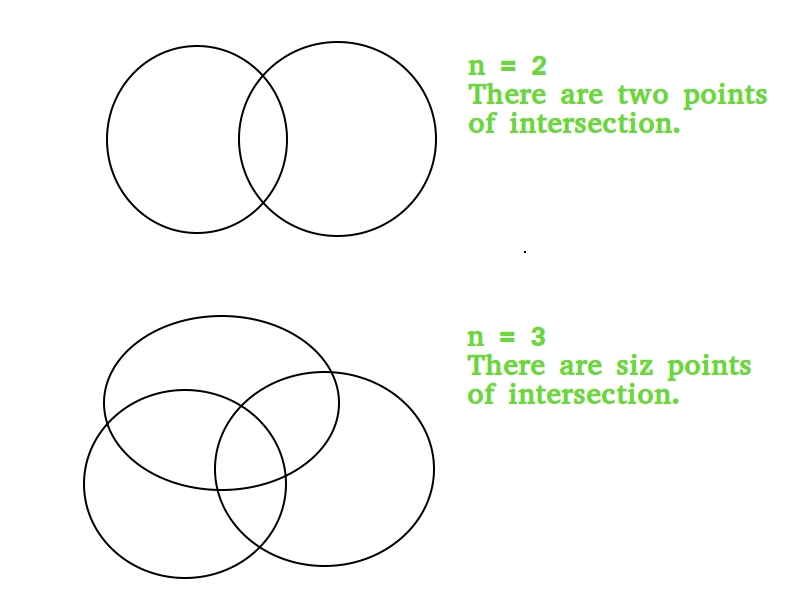GeeksforGeeks App
Open AppBrowser
Continue

# Maximum points of intersection n circles

Given a number n, we need to find the maximum number of times n circles intersect.

Examples:

Input :  n = 2
Output : 2

Input :  n = 3
Output : 6## Description and Derivation

As we can see in above diagram, for each pair of circles, there can be maximum two intersection points. Therefore if we have n circles then there can be nC2 pairs of circles in which each pair will have two intersections. So by this, we can conclude that by looking at all possible pairs of circles the mathematical formula can be made for the maximum number of intersections by n circles is given by 2 * nC2
2 * nC2 = 2 * n * (n – 1)/2 = n * (n-1)

## C++

 `// CPP program to find maximum number of``// intersections of n circles``#include ``using` `namespace` `std;` `// Returns maximum number of intersections``int` `intersection(``int` `n)``{``   ``return` `n * (n - 1);``}` `int` `main()``{``    ``cout << intersection(3) << endl;``    ``return` `0;``}``// This code is contributed by``// Manish Kumar Rai.`

## Java

 `// Java program to find maximum number of``// intersections of n circles``import` `java.io.*;` `public` `class` `GFG {` `    ``// for the calculation of 2*(nC2)``    ``static` `int` `intersection(``int` `n)``    ``{``       ``return` `n * (n - ``1``);``    ``}` `    ``public` `static` `void` `main(String[] args) ``throws` `IOException``    ``{``        ``System.out.println(intersection(``3``));``    ``}``}``// This code is contributed by``// Manish Kumar Rai`

## Python3

 `# python program to find maximum number of``# intersections of n circles``# Returns maximum number of intersections``def` `intersection(n):``    ` `   ``return` `n ``*` `(n ``-` `1``);` `# Driver code``print``(intersection(``3``))` `# This code is contributed by Sam007`

## C#

 `// C# program to find maximum number of``// intersections of n circles``using` `System;``class` `GFG {` `    ``// for the calculation of 2*(nC2)``    ``static` `int` `intersection(``int` `n)``    ``{``        ``return` `n * (n - 1);``    ``}` `// Driver Code``public` `static` `void` `Main()``{``    ``Console.WriteLine(intersection(3));``}` `}` `// This code is contributed by Sam007`

## PHP

 ``

## Javascript

 ``

Output:

`6`

Time Complexity: O(1)
Auxiliary Space: O(1)

My Personal Notes arrow_drop_up